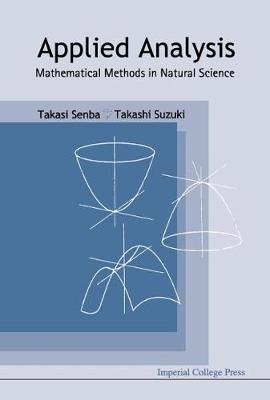•# Applied Analysis: Mathematical Methods In Natural Science (Hardback)

(author), (author)
£91.00
Hardback 392 Pages / Published: 16/04/2004
• We can order this

Usually dispatched within 3 weeks

This book provides a general introduction to applied analysis; vector analysis with physical motivation, calculus of variation, Fourier analysis, eigenfunction expansion, distribution, and so forth, including a catalogue of mathematical theories, such as basic analysis, topological spaces, complex function theory, real analysis, and abstract analysis. This book also uses fundamental ideas of applied mathematics to discuss recent developments in nonlinear science, such as mathematical modeling of reinforced random motion of particles, semiconductor device equation in applied physics, and chemotaxis in biology. Several tools in linear PDE theory, such as fundamental solutions, Perron's method, layer potentials, and iteration scheme, are described, as well as systematic descriptions on the recent study of the blowup of the solution.

Publisher: Imperial College Press
ISBN: 9781860944406
Number of pages: 392
Weight: 671 g
Dimensions: 230 x 160 x 25 mm